C Program to find Area & Circumference of Circle

In this tutorial you will learn about the C Program to find Area & Circumference of Circle and its application with practical example.

C Program to find Area & Circumference of Circle

In this tutorial, we will learn to create a C program that will find the Area & Circumference of Circle in C programming.

Prerequisites

Before starting with this tutorial we assume that you are best aware of the following C programming topics:

• Operators in C Programming.
• Basic Input and Output function in C Programming.
• Basic C programming.
• While loop in C programming.

Program to find Area & Perimeter of Circle:-

As we all know the c is a very powerful language. With the help of c programming language, we can make many programs. We can perform many input-output operations using c programming. In today’s tutorial, we take the input in the form radius of the Circle. Then we will find its area and Circumference with the help of the c programming language.

With the help of this program, we can find the Area & Circumference of the Circle.

Output:-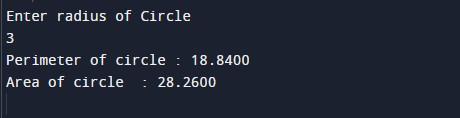In the above program, we have first initialized the required variable.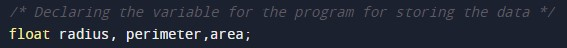• radius = it will hold the float value.
• area = it will hold the float value.
• perimeter = it will hold the float value.

Taking the input from the message.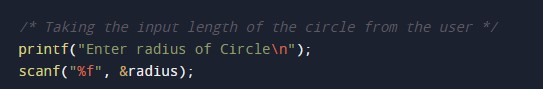Calculating the Area of Circle.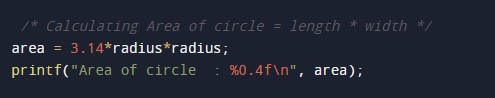Calculating the Perimeter of Circle.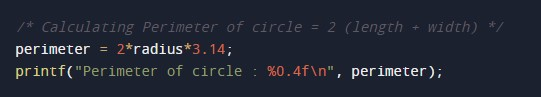In this tutorial we have learn about the C Program to find Area & Circumference of Circle and its application with practical example. I hope you will like this tutorial.